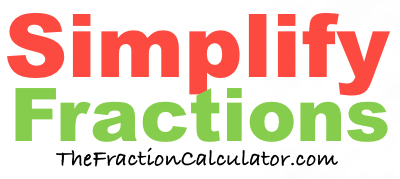What is 5/4 simplified?Here we will simplify 5/4 to its simplest form and convert it to a mixed number if necessary.

In the fraction 5/4, 5 is the numerator and 4 is the denominator.

When you ask "What is 5/4 simplified?", we assume you want to know how to simplify the numerator and denominator to their smallest values, while still keeping the same value of the fraction.

We do this by first finding the greatest common factor of 5 and 4, which is 1.

Then, we divide both 5 and 4 by the greatest common factor to get the following simplified fraction:

5/4

Therefore, this equation is true:

5/4 = 5/4

If the numerator is greater than or equal to the denominator of a fraction, then it is called an improper fraction. In that case, you could convert it into a whole number or mixed number fraction.

5/4 = 1 1/4

Simplify Fractions
Here you can submit another fraction for us to simplify:

/

What is 5/5 simplified?
Here is the next fraction on our list that we simplified.